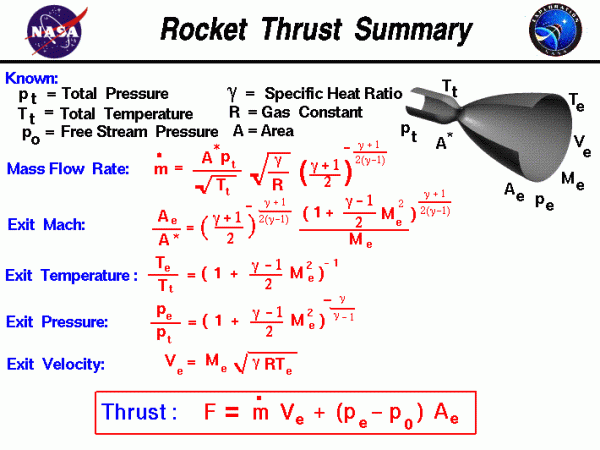# Thrust Equations Summary## Thrust

On this slide, we have collected all of the equations necessary to calculate the thrust of a rocket engine. In a rocket engine, stored fuel and stored oxidizer are ignited in a combustion chamber. The combustion produces great amounts of exhaust gas at high temperature and pressure. The hot exhaust is passed through a nozzle which accelerates the flow. Thrust is produced according to Newton’s third law of motion.

### What determines the amount of Thrust?

The amount of thrust produced by the rocket depends on the mass flow rate through the engine, the exit velocity of the exhaust, and the pressure at the nozzle exit. All of these variables depend on the design of the nozzle. The smallest cross-sectional area of the nozzle is called the throat of the nozzle. The hot exhaust flow is choked at the throat, which means that the Mach number is equal to 1.0 in the throat and the mass flow rate m dot ($$\bf \dot{m}$$) is determined by the throat area.

$$\LARGE \dot{m}=\frac{A^{*}p_{t}}{\sqrt{T_{t}}}\sqrt{\frac{\gamma}{R}}(\frac{\gamma+1}{2})^{-\frac{\gamma+1}{2(\gamma-1)}}$$

where A* is the area of the throat, pt is the total pressure in the combustion chamber, Tt is the total temperature in the combustion chamber, gam ($$\bf \gamma$$) is the ratio of specific heats of the exhaust, and R is the gas constant.

The area ratio from the throat to the exit Ae sets the exit Mach number:

$$\LARGE \frac{A_{e}}{A^{*}}=(\frac{\gamma+1}{2})^{-\frac{\gamma+1}{2(\gamma-1)}}\frac{(1+\frac{\gamma-1}{2}M_{e}^{2})^{\frac{\gamma+1}{2(\gamma-1)}}}{M_{e}}$$

We can determine the exit pressure pe and exit temperature Te from the isentropic relations at the nozzle exit:

$$\LARGE \frac{p_{e}}{p_{t}}=(1+\frac{\gamma-1}{2}M_{e}^{2})^{-\frac{\gamma}{\gamma-1}}$$

$$\LARGE \frac{T_{e}}{T_{t}}=(1+\frac{\gamma-1}{2}M_{e}^{2})^{-1}$$

Knowing Te we can use the equation for the speed of sound and the definition of the Mach number to calculate the exit velocity Ve:

$$\LARGE V_{e}=M_{e}\sqrt{\gamma RT_{e}}$$

We now have all the information necessary to determine the thrust of a rocket. The exit pressure is only equal to free stream pressure at some design condition. We must, therefore, use the longer version of the generalized thrust equation to describe the thrust of the system. If the free stream pressure is given by p0, the rocket thrust equation is given by:

$$\LARGE F=\dot{m}V_{e}+A_{e}(p_{e}-p_{0})$$

The thrust equation shown above works for both liquid rocket and solid rocket engines. There is also an efficiency parameter called the specific impulse which works for both types of rockets and greatly simplifies the performance analysis for rockets.

You can explore the design and operation of a rocket nozzle with our interactive thrust simulator program which runs on your browser.

Provide feedback